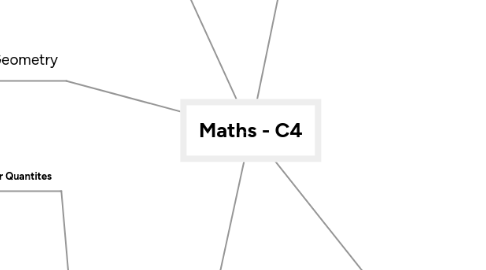# Maths - C4

Get Started. It's FreeMaths - C4## 1. Differentiation

### 1.1. Trigonometric functions

1.1.1. dy/dx sinx=cosx dy/dx sin(ax+b)=acos(ax+b)

1.1.2. dy/dx cosx=-sinx dy/dx cos(ax+b)=-asin(ax+b)

1.1.3. dy/dx tanx = sec^2x dy/dx tan(ax+b)=asec^2(ax+b)

1.1.4. Chain rule comes in mighty handy with this

### 1.2. Parametric Functions

1.2.1. If x and y are each expressed in terms of a parameter, t: dy/dx = dy/dt x dt/dx

### 1.3. Implicit Functions

1.3.1. To find dy/dx when an equation in x and y is given implicitly, differentiate each term in respect to X

1.3.2. d/dx(f(y)) = d/dy(f(y)) x dy/dx

## 2. Intergration

### 2.1. Trigonometric Functions

2.1.1. ∫cosx dx=sinx+c ∫cos(ax+b) dx=(1/a)sin(ax+b)+c ∫sinx dx=-cosx+c ∫sin(ax+b) dx=-(1/a)cos(ax+b)+c ∫sec^2x dx=tanx +c ∫sec^2(ax+b)=(1/a)tan(ax+b)+c

2.1.2. ∫sin^n(x)cosx dx=1/n+1(sin^n+1(x)+c) ∫cos^n(x)sinx dx =-1/n+1(cos^n+1(x)+c)

2.1.3. For odd powers of n, split the function in to even powers or one, then use trig identities to simplify the function into something recognisable and work from there

2.1.4. To integrate even powers use the double angle identities: cos^2(x)=1/2(1+cos2x) sin^2(x)=1/2(1-cos2x)

### 2.2. Integrals Leading to a Logarithmic Function

2.2.1. ∫f'(x)/f(x) dx= ln|f(x)|+c

2.2.2. You will need to know this in order to help find the area under a curve

### 2.3. Partial Fractions

2.3.1. One of the reasons why we learn partial fractions is so that we can integrate them; so we would split the fraction and integrate as usual

### 2.4. Substitution

2.4.1. Sometimes an integral is made easier by using a substitution.

2.4.2. ∫f(x) dx=∫f(x)(dx/du) du

2.4.3. When doing this remember to change the x limits to u limits as soon as possible - it helps

2.4.4. A general result through this is: ∫f'(x)[f(x))]^n dx=(1/n+1)[f(x)]^(n+1) + c

### 2.5. Integration By Parts

2.5.1. Another way of integrating is integrating through parts

2.5.2. ∫(u)(dv/dx)dx=uv-∫(v)(du/dx)dx

2.5.3. Sometimes you won't get the answer straight after putting it in the formula so put it in again.

2.5.4. Always make U the simplest variable or ln(x)

2.5.5. Also under definite integrals the rules are the same, don't act to smart, if you get confused go back to the basics!

### 2.6. Differential Equations

2.6.1. When integrating, we get a general solution, sometimes we may get given points in a graph in order to work out c, to get a particular solution

2.6.2. A differential equation can be written as: f(y)(dy/dx)=g(x) this can be solved be separating the variables where: ∫f(y) dy = ∫g(x) dx

### 2.7. Exponential Growth and Decay

2.7.1. You may have to form a differential equation from given information; this is normally exponential growth or decay

2.7.2. Exponential growth: dy/dx = ky so y=Ae^-kt Examples include population growth

2.7.3. Exponential decay: dy/dx = -ky so y=Ae^-kt Examples include the disintegration of radioactive materials and Newton's law of cooling

## 3. Vectors

### 3.1. Vector and Scalar Quantites

3.1.1. A scalar quantity has a size (magnitude) and no direction

3.1.2. A vector quantity has both magnitude and a direction

### 3.2. Component Form

3.2.1. When working in two dimension, it is quite useful to express a vector in terms of i and j. They are unit vector and can be written as r=ai+bj

3.2.2. In three dimension a third vector (k) is used to represent a unit vector in the z-axis

3.2.3. This form can also be used to represent position vectors

### 3.3. Finding the Magnitude of a Vector

3.3.1. If r = ai+bj+ck then |r|= √(a^2+b^2+c^2 )

### 3.4. Scalar Product

3.4.1. a.b=|a||b|cosθ

3.4.2. If two vectors are parallel then θ=0 so a.b=|a||b|

3.4.3. If two vectors are perpendicular then θ=90 so a.b=0

### 3.5. Vector Equation of a Line

3.5.1. R is the position vector of any point on the line B is the direction vector of the line (b-a) A is any point on the line t is the scalar parameter (constant or so to say)

3.5.2. R= A+tB

### 3.6. Pairs of Lines

3.6.1. In 2-D, a pair of lines either are parallel or the intersect

3.6.2. In 3-D, a pair of lines either are parallel, they intersect or they are skew

3.6.3. Parallel = t is a multiple of the other Intersect = there is a unique value for t Skew = no unique solution, nor a multiple, completely unrelated values of t

## 4. Coordinate Geometry

### 4.1. Cartesian Form

4.1.1. A curve in Cartesian form is written in terms of x and y

4.1.2. To sketch a graph in Cartesian from: 1. Check for symmetry (if the powers are even) 2. Find where the curves cross the axis (x=0;y=0) 3. Check for Stationary points (dy/dx = 0) 4. Check what happens for large values of x and y 5. Check for any discontinuities

### 4.2. Parametric Form

4.2.1. A curve in Parametric form can be expressed in x, y and in a third variable, for example: t.

4.2.2. You will usually be asked to turn it in to Cartesian form (Make t the subject and sub it into the other equation) and then to draw a graph for it

4.2.3. As this is a synoptic unit, they may bring in other topics into this, most likely trigonometry (Pythagoras Identities) So get some practise in using them

## 5. Algebra And Series

### 5.1. Rational Expression

5.1.1. Can be in the form of f(x)/g(x)

5.1.2. To simplify, just factorise the two and eliminate like terms

5.1.3. When adding or subtracting, just use the lowest common denominator

5.1.4. Not that hard, but ever so long so don't be lazy and do it!!

### 5.2. Partial Fractions

5.2.1. This is splitting fractions into two or even three is required; and of course you have to work out the numerator for each new fraction

5.2.2. Only proper fractions can be split, if it is improper, then you must turn it into a mixed fraction before moving forward

### 5.3. Binomial Expansion For Rational, n

5.3.1. (1+x)^n can be written through the binomial expansion formula

5.3.2. The formula is given, but learn how to manipulate the formula algebraically as it will help.